# Texas Go Math Grade 4 Lesson 5.3 Answer Key Subtract Fractions Using Models

Refer to our Texas Go Math Grade 4 Answer Key Pdf to score good marks in the exams. Test yourself by practicing the problems from Texas Go Math Grade 4 Lesson 5.3 Answer Key Subtract Fractions Using Models.

## Texas Go Math Grade 4 Lesson 5.3 Answer Key Subtract Fractions Using Models

Essential Question

How can you subtract fractions with like denominators using models?
We can directly subtract the numerators
if there is like denominators using models

Unlock the ProblemA rover needs to travel $$\frac{5}{8}$$ mile to reach its destination. It has already traveled $$\frac{3}{8}$$ mile. How much farther does the rover need to travel?

Compare fractions to find the difference.

Shade the model to show the total distance
Then shade the model to show how much distance the rover has already covered.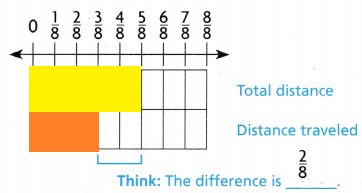Explanation:
$$\frac{5}{8}$$ – $$\frac{3}{8}$$ = $$\frac{2}{8}$$

STEP 2 Write the difference.
$$\frac{5}{8}-\frac{3}{8}$$ = $$\frac{}{8}$$
So, the rover needs to travel __________ mile farther.
$$\frac{5}{8}-\frac{3}{8}$$ = $$\frac{2}{8}$$
So, the rover needs to travel $$\frac{2}{8}$$ mile farther.

Another Way Use fraction strips.Use five $$\frac{1}{8}$$-size parts to model the whole distance.
How many $$\frac{1}{8}$$-size parts should you cross out to
model the distance the rover has traveled? ___________
How many $$\frac{1}{8}$$-size parts are left? ___________
Write the difference.
$$\frac{5}{8}$$ – __________ = _________
How many $$\frac{1}{8}$$-size parts should you cross out to
model the distance the rover has traveled? 3 we have to cross out.
How many $$\frac{1}{8}$$-size parts are left? 2 are left
Write the difference.
$$\frac{5}{8}$$ – $$\frac{3}{8}$$ = $$\frac{2}{8}$$

Share and Show

Lisa needs $$\frac{4}{5}$$ pound of shrimp to make shrimp salad. She has $$\frac{1}{5}$$ pound of shrimp. How much more shrimp does Lisa need to make the salad?
Subtract $$\frac{4}{5}-\frac{1}{5}$$. Use the model to help.
Shade the model to show how much shrimp Lisa needs.
Then shade the model to show how much shrimp Lisa has.
Compare the difference between the two shaded rows.
$$\frac{4}{5}-\frac{1}{5}$$ = $$\frac{}{5}$$ pound
Lisa needs __________ pound more shrimp.Explanation:
Shaded $$\frac{4}{5}$$  total to travel
$$\frac{1}{5}$$ shaded which was travelled
$$\frac{4}{5}-\frac{1}{5}$$ = $$\frac{3}{5}$$ pound
Lisa needs $$\frac{3}{5}$$ pound more shrimp.

Use the model to find the difference.

Question 2.
$$\frac{3}{6}-\frac{2}{6}$$ = $$\frac{}{6}$$$$\frac{3}{6}-\frac{2}{6}$$ = $$\frac{1}{6}$$
Explanation:
As the denominators are same we can directly
Subtract the numerators in the fractions

Question 3.
$$\frac{8}{10}-\frac{3}{10}$$ = $$\frac{}{10}$$$$\frac{8}{10}-\frac{3}{10}$$ = $$\frac{5}{10}$$
Explanation:
As the denominators are same we can directly
Subtract the numerators in the fractions

Subtract. Use models to help.

Question 4.
$$\frac{5}{8}-\frac{2}{8}$$ = __________$$\frac{5}{8}-\frac{2}{8}$$ = $$\frac{3}{8}$$
Explanation:
As the denominators are the same we can directly
Subtract the numerators in the fractions

$$\frac{7}{12}-\frac{2}{12}$$ = __________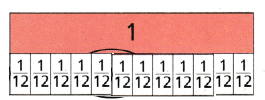$$\frac{7}{12}-\frac{2}{12}$$ =  $$\frac{5}{12}$$
Explanation:
As the denominators are same we can directly
Subtract the numerators in the fractions

Question 6.
$$\frac{3}{4}-\frac{2}{4}$$ = __________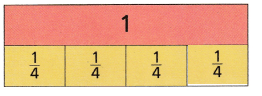$$\frac{3}{4}-\frac{2}{4}$$ = $$\frac{1}{4}$$
Explanation:
As the denominators are same we can directly
Subtract the numerators in the fractions

Math Talk

Mathematical Processes
Explain why the numerator changes when you subtract fractions with like denominators, but the denominator doesn’t.
Explanation:
The denominator defines total number of pieces or total amount of the whole
So, Numerator changes but denominator doesn’t change.

Problem Solving

Question 7.
HOT. Explain how you could find the unknown addend in $$\frac{2}{6}$$ + _______ = 1 without using a model.
$$\frac{2}{6}$$ + $$\frac{4}{6}$$= 1
Explanation:
To make the whole how much we have to add
1 – $$\frac{2}{6}$$ = $$\frac{4}{6}$$
so, we have to add $$\frac{4}{6}$$

Danis bean plant grew $$\frac{6}{8}$$ inch the first week and $$\frac{2}{8}$$ inch the second week. How much less did Dani’s plant grow the second week?
$$\frac{6}{8}$$ – $$\frac{2}{8}$$  = $$\frac{4}{8}$$
Explanation:
Danis bean plant grew $$\frac{6}{8}$$ inch the first week
and $$\frac{2}{8}$$ inch the second week.
$$\frac{6}{8}$$ – $$\frac{2}{8}$$  = $$\frac{4}{8}$$
Dani’s plant grow less $$\frac{4}{8}$$  the second week

Unlock the Problem

Question 9.
H.O.T. Reasoning Multi-Step Mrs. Ruiz served a pie for dessert two nights in a row. The drawings below show the pie after her family ate dessert on each night. What fraction of the pie did they eat on the second night?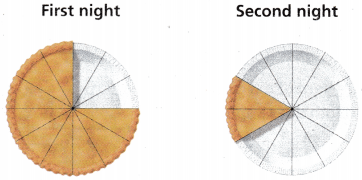(A) $$\frac{2}{12}$$
(B) $$\frac{5}{12}$$
(C) $$\frac{7}{12}$$
(D) $$\frac{10}{12}$$
Explanation:
$$\frac{10}{12}$$ is fraction of the pie  they eat on the second night

a. What do you need to know?
How much they ate on the second night

b. How can you find the number of pieces eaten on the second night?
Total – what part they have eaten

c. Explain the steps you used to solve the problem.
1 – $$\frac{2}{12}$$  = $$\frac{10}{12}$$

d. Complete the sentences.
After the first night, _______ pieces were left.
After the second night, _______ pieces were left.
So, _______ of the pie was eaten on the second night.
After the first night, $$\frac{3}{12}$$  pieces were left.
After the second night, $$\frac{2}{12}$$ pieces were left.
So, $$\frac{10}{12}$$ of the pie was eaten on the second night.

e. Fill in the bubble for the correct answer choice above.
$$\frac{10}{12}$$  is the correct answer

Angel is climbing a mountain. Ile has already climbed $$\frac{3}{5}$$ of the way. What fraction of the way does Angel have left to climb?(A) $$\frac{2}{5}$$
(B) $$\frac{1}{2}$$
(C) $$\frac{3}{5}$$
(D) $$\frac{2}{3}$$
Explanation:
He has already climbed $$\frac{3}{5}$$
1 – $$\frac{3}{5}$$ = $$\frac{2}{5}$$

Question 11.
Sophia has $$\frac{7}{8}$$ yard of blue cloth and $$\frac{3}{8}$$ yard of red cloth to make a kite. How much more blue cloth does Sophia have than red cloth?(A) $$\frac{3}{8}$$ yard
(B) $$\frac{4}{8}$$ yard
(C) $$\frac{5}{8}$$ yard
(D) $$\frac{10}{8}$$ yard
Explanation:
$$\frac{7}{8}$$ – $$\frac{3}{8}$$  = $$\frac{4}{8}$$
$$\frac{4}{8}$$ more blue cloth does Sophia have than red cloth

Question 12.
Multi-Step David buys $$\frac{3}{4}$$ pound of bananas. Molly buys $$\frac{1}{4}$$ pound less of bananas than David. Jude buys $$\frac{1}{4}$$ pound less of bananas than Molly. How many pounds of bananas does Jude buy?
(A) $$\frac{2}{4}$$ pound
(B) $$\frac{1}{3}$$ pound
(C) $$\frac{1}{4}$$ pound
(D) $$\frac{1}{2}$$ pound
Explanation:
$$\frac{3}{4}$$ – $$\frac{1}{4}$$ = $$\frac{2}{4}$$
$$\frac{2}{4}$$ pounds of bananas does Jude buy.

Judi ate $$\frac{7}{8}$$ of a small pizza and Jack ate $$\frac{2}{8}$$ of a second small pizza. How much more of a pizza did Judi eat?
(A) $$\frac{8}{8}$$
(B) 1
(C) $$\frac{6}{8}$$
(D) $$\frac{5}{8}$$
Explanation:
$$\frac{7}{8}$$ – $$\frac{2}{8}$$ = $$\frac{5}{8}$$
$$\frac{5}{8}$$ more of a pizza that Judi eat

### Texas Go Math Grade 4 Lesson 5.3 Homework and Practice Answer Key

Use the model to find the difference.

Question 1.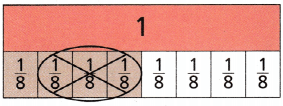$$\frac{4}{8}-\frac{3}{8}$$ = ___________
$$\frac{4}{8}-\frac{3}{8}$$ = $$\frac{1}{8}$$
Explanation:
As the denominators are same we can directly
Subtract the numerators in the fractions

Question 2.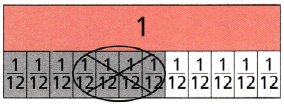$$\frac{7}{12}-\frac{4}{12}$$ = ___________
$$\frac{7}{12}-\frac{4}{12}$$ = $$\frac{3}{12}$$
Explanation:
As the denominators are the same we can directly
Subtract the numerators in the fractions

Subtract. Use models to help.

$$\frac{5}{7}-\frac{2}{7}$$ = ___________
$$\frac{5}{7}-\frac{2}{7}$$ = $$\frac{3}{7}$$
Explanation:
As the denominators are the same we can directly
Subtract the numerators in the fractions

Question 4.
$$\frac{4}{5}-\frac{1}{5}$$ = ___________
$$\frac{4}{5}-\frac{1}{5}$$ = $$\frac{3}{5}$$
Explanation:
As the denominators are same we can directly
Subtract the numerators in the fractions

Question 5.
$$\frac{5}{6}-\frac{3}{6}$$ = ___________
$$\frac{5}{6}-\frac{3}{6}$$ = $$\frac{2}{6}$$
Explanation:
As the denominators are same we can directly
Subtract the numerators in the fractions

Question 6.
$$\frac{8}{10}-\frac{3}{10}$$ = ___________
$$\frac{8}{10}-\frac{3}{10}$$ = $$\frac{5}{10}$$
Explanation:
As the denominators are same we can directly
Subtract the numerators in the fractions

Problem Solving

Question 7.
Martin’s house is $$\frac{6}{8}$$ mile from his school. If he walks $$\frac{4}{8}$$ mile, how much further does he need to walk before he reaches the school? Draw a model to find the difference.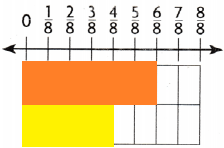Explanation:
$$\frac{6}{8}$$ – $$\frac{4}{8}$$ = $$\frac{2}{8}$$
$$\frac{2}{8}$$  he need to walk before he reaches the school

Go Math Lesson 5.3 Practice and Homework 4th Grade Question 8.
Cynthia is mowing a yard. She has already mowed $$\frac{3}{4}$$ of the yard. What fraction of the yard does Cynthia have left to mow? Draw a model to find the difference.
1 – $$\frac{3}{4}$$ = $$\frac{1}{4}$$
Explanation:
Drawn the fraction strip modelLesson Check

Question 9.
Cheri used $$\frac{5}{10}$$ yard of ribbon to make abow and $$\frac{2}{10}$$ yard of ribbon to make a bookmark. How much more ribbon did she use for the bow than she did for the bookmark?
(A) $$\frac{7}{10}$$
(B) $$\frac{4}{10}$$
(C) $$\frac{5}{10}$$
(D) $$\frac{3}{10}$$
Explanation:
$$\frac{5}{10}$$ – $$\frac{2}{10}$$  = $$\frac{3}{10}$$
$$\frac{3}{10}$$ more ribbon that she use for the bow than she did for the bookmark

Question 10.
Carl had $$\frac{7}{12}$$ of his cake left after his birthday. Then he and his friend ate $$\frac{2}{12}$$ of the cake. how much of the cake is left now?
(A) $$\frac{5}{12}$$
(B) $$\frac{9}{12}$$
(C) $$\frac{6}{12}$$
(D) $$\frac{4}{12}$$
Explanation:
$$\frac{7}{12}$$ – $$\frac{2}{12}$$ = $$\frac{5}{12}$$
$$\frac{5}{12}$$ of the cake is left now

Question 11.
Carson needs $$\frac{4}{5}$$ gallon of paint for his project. He has $$\frac{1}{5}$$ gallon of paint. How much more paint does Carson need for his project?
(A) $$\frac{5}{5}$$ gallon
(B) $$\frac{2}{5}$$ ga1lon
(C) $$\frac{3}{5}$$ gallon
(D) $$\frac{4}{5}$$ gallon
Explanation:
$$\frac{4}{5}$$ – $$\frac{1}{5}$$ = $$\frac{3}{5}$$ gallon
$$\frac{3}{5}$$ gallon more paint does Carson need for his project

Hannah needs to read $$\frac{5}{6}$$ of a hook by Friday to stay on schedule. She has read $$\frac{3}{6}$$ of the book so far, What fraction of the book does Hannah still need to read?
(A) $$\frac{4}{6}$$
(B) $$\frac{3}{6}$$
(C) $$\frac{8}{6}$$
(D) $$\frac{2}{6}$$
Explanation:
$$\frac{5}{6}$$ – $$\frac{3}{6}$$ = $$\frac{2}{6}$$
$$\frac{2}{6}$$ fraction of the book that Hannah still need to read

Question 13.
Multi-Step Mrs. Gray sent $$\frac{5}{8}$$ of her class to the library and $$\frac{2}{8}$$ of her class to the computer lab. What fraction of the class remained in the classroom?
(A) $$\frac{4}{8}$$
(B) $$\frac{1}{8}$$
(C) $$\frac{7}{8}$$
(D) $$\frac{3}{8}$$
Explanation:
$$\frac{5}{8}$$ + $$\frac{2}{8}$$ = $$\frac{7}{8}$$
1 – $$\frac{7}{8}$$ = $$\frac{1}{8}$$
$$\frac{1}{8}$$ fraction of the class remained in the classroom

Go Math Lesson 5.3 Homework Answer Key Question 14.
Multi-Step Jarrod planted beans in $$\frac{3}{5}$$ of his garden. He planted LomaEnes in $$\frac{1}{5}$$ of his garden. What part of the garden does not have tomatoes or beans?
(A) $$\frac{1}{5}$$
(B) $$\frac{2}{5}$$
(C) $$\frac{3}{5}$$
(D) $$\frac{4}{5}$$
$$\frac{3}{5}$$ + $$\frac{1}{5}$$  = $$\frac{4}{5}$$
1 – $$\frac{4}{5}$$ = $$\frac{1}{5}$$
$$\frac{1}{5}$$ part of the garden does not have tomatoes or beans